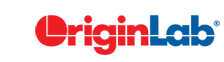# 18.13.1 2D Wavelet Decomposition

This function performs single-level 2D wavelet decomposition with the chosen wavelet decomposition filters. The result is comprised of four matrices: the approximation coefficients, the horizontal details coefficients, the vertical details coefficients, and the diagonal details coefficients.

The decomposition filters are determined by the chosen wavelet. Currently, three types of wavelets are available, including Haar, Daubechies ($N = 2,3,4,5,6,7,8,9,10$), and Biorthogonal ($Nr.Nd=1.1,1.3,1.5,2.2,2.4,2.6,3.1,3.3,3.5,3.7$).

Note that the end extension method for wavelet decomposition is always assumed to be periodic.

To use 2D wavelet decomposition:

1. Make a matrix book active.
2. Select Analysis: Signal Processing: Wavelet: 2D Decompose from the Origin menu.
The DWT2 Dialog Box
Algorithm
Reference